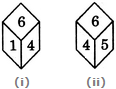# Non Verbal Reasoning - Cubes and Dice - Discussion

### Discussion :: Cubes and Dice - Section 2 (Q.No.18)

18.

Two positions of a dice are shown below. When number 1 is on the top, what number will be at the bottom?[A]. 2 [B]. 3 [C]. 5 [D]. Cannot be determined

Explanation:

Number 6 is common to both the positions of the dice. We assume the dice in fig. (ii) to be rotated so that 6 remains on the top face (i.e. face V as per activity 1) and the number 4 in fig. (ii) moves to the FR-RH face (i.e. face I) as in fig. (i), then 5 will move to the RR-RH face (i.e. face II). Clearly, 5 (which lies on face II) and 1 (which lies on face IV) will be opposite to each other. So, when 1 is on the top, then 5 will be at the bottom.